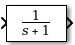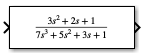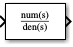# Transfer Fcn

Model linear system by transfer function

• Library:

•## Description

The Transfer Fcn block models a linear system by a transfer function of the Laplace-domain variable `s`. The block can model single-input single-output (SISO) and single-input multiple-output (SIMO) systems.

### Conditions for Using This Block

The Transfer Fcn block assumes the following conditions:

• The transfer function has the form

`$H\left(s\right)=\frac{y\left(s\right)}{u\left(s\right)}=\frac{num\left(s\right)}{den\left(s\right)}=\frac{num\left(1\right){s}^{nn-1}+num\left(2\right){s}^{nn-2}+\dots +num\left(nn\right)}{den\left(1\right){s}^{nd-1}+den\left(2\right){s}^{nd-2}+\dots +den\left(nd\right)},$`

where u and y are the system input and outputs, respectively, nn and nd are the number of numerator and denominator coefficients, respectively. num(s) and den(s) contain the coefficients of the numerator and denominator in descending powers of s.

• The order of the denominator must be greater than or equal to the order of the numerator.

• For a multiple-output system, all transfer functions have the same denominator and all numerators have the same order.

### Modeling a Single-Output System

For a single-output system, the input and output of the block are scalar time-domain signals. To model this system:

1. Enter a vector for the numerator coefficients of the transfer function in the Numerator coefficients field.

2. Enter a vector for the denominator coefficients of the transfer function in the Denominator coefficients field.

### Modeling a Multiple-Output System

For a multiple-output system, the block input is a scalar and the output is a vector, where each element is an output of the system. To model this system:

1. Enter a matrix in the Numerator coefficients field.

Each row of this matrix contains the numerator coefficients of a transfer function that determines one of the block outputs.

2. Enter a vector of the denominator coefficients common to all transfer functions of the system in the Denominator coefficients field.

### Specifying Initial Conditions

A transfer function describes the relationship between input and output in Laplace (frequency) domain. Specifically, it is defined as the Laplace transform of the response (output) of a system with zero initial conditions to an impulse input.

Operations like multiplication and division of transfer functions rely on zero initial state. For example, you can decompose a single complicated transfer function into a series of simpler transfer functions. Apply them sequentially to get a response equivalent to that of the original transfer function. This will not be correct if one of the transfer functions assumes a non-zero initial state. Furthermore, a transfer function has infinitely many time domain realizations, most of whose states do not have any physical meaning.

For these reasons, Simulink® presets the initial conditions of the Transfer Fcn block to zero. To specify initial conditions for a given transfer function, convert the transfer function to its controllable, canonical state-space realization using `tf2ss` . Then, use the State-Space block. The `tf2ss` utility provides the `A`, `B`, `C`, and `D` matrices for the system.

For more information, type `help tf2ss` or see the Control System Toolbox™ documentation.

### Transfer Function Display on the Block

The Transfer Fcn block displays the transfer function depending on how you specify the numerator and denominator parameters.

• If you specify each parameter as an expression or a vector, the block shows the transfer function with the specified coefficients and powers of s. If you specify a variable in parentheses, the block evaluates the variable.

For example, if you specify Numerator coefficients as `[3,2,1]` and Denominator coefficients as `(den)`, where `den` is `[7,5,3,1]`, the block looks like this:• If you specify each parameter as a variable, the block shows the variable name followed by `(s)`.

For example, if you specify Numerator coefficients as `num` and Denominator coefficients as `den`, the block looks like this:## Ports

### Input

expand all

Input signal, specified as a scalar with data type `double`.

Data Types: `double`

### Output

expand all

Output signal, provided as a scalar or vector with data type `double`.

• For a single-output system, the input and output of the block are scalar time-domain signals.

• For a multiple-output system, the input is a scalar, and the output is a vector, where each element is an output of the system.

Data Types: `double`

## Parameters

expand all

Define the numerator coefficients of the transfer function.

• For a single-output system, enter a vector for the numerator coefficients of the transfer function.

• For a multiple-output system, enter a matrix. Each row of this matrix contains the numerator coefficients of a transfer function that determines one of the block outputs.

#### Programmatic Use

 Block Parameter: `Numerator` Type: character vector, string Values: vector | matrix Default: `''`

Define the row vector of denominator coefficients.

• For a single-output system, enter a vector for the denominator coefficients of the transfer function.

• For a multiple-output system, enter a vector containing the denominator coefficients common to all transfer functions of the system.

#### Programmatic Use

 Block Parameter: `Denominator` Type: character vector, string Values: vector Default: `'[1 1]'`

Absolute tolerance for computing block states, specified as a positive, real-valued, scalar or vector. To inherit the absolute tolerance from the Configuration Parameters, specify `auto` or `-1`.

• If you enter a real scalar, then that value overrides the absolute tolerance in the Configuration Parameters dialog box for computing all block states.

• If you enter a real vector, then the dimension of that vector must match the dimension of the continuous states in the block. These values override the absolute tolerance in the Configuration Parameters dialog box.

• If you enter `auto` or –1, then Simulink uses the absolute tolerance value in the Configuration Parameters dialog box (see Solver Pane) to compute block states.

#### Programmatic Use

 Block Parameter: ` AbsoluteTolerance` Type: character vector, string Values: `'auto'` | `'-1'` | any positive real-valued scalar or vector Default: `'auto'`

Assign a unique name to each state. If this field is blank (`' '`), no name assignment occurs.

• To assign a name to a single state, enter the name between quotes, for example, `'position'`.

• To assign names to multiple states, enter a comma-delimited list surrounded by braces, for example, `{'a', 'b', 'c'}`. Each name must be unique.

• To assign state names with a variable in the MATLAB® workspace, enter the variable without quotes. A variable can be a character vector, string, cell array, or structure.

#### Limitations

• The state names apply only to the selected block.

• The number of states must divide evenly among the number of state names.

• You can specify fewer names than states, but you cannot specify more names than states.

For example, you can specify two names in a system with four states. The first name applies to the first two states and the second name to the last two states.

#### Programmatic Use

 Block Parameter: `ContinuousStateAttributes` Type: character vector, string Values: `' '` | user-defined Default: `' '`

## Block Characteristics

 Data Types `double` Direct Feedthrough `yes` Multidimensional Signals `no` Variable-Size Signals `no` Zero-Crossing Detection `no`